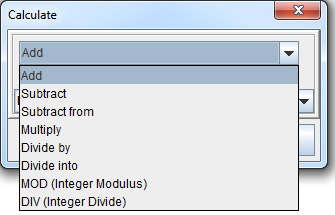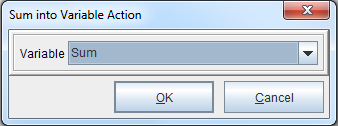## Latest News

the latest news from our team

# Calculation and Sum Procedures

Calculation and Sum are procedures that are often used together. For instance, a process in which Calculation is used to multiply a set of values and Sum is used to add the total to a separate variable. Such a process is detailed here: Math Calculations with Arrays

## Calculation

Calculation implements numeric math calculations/math functions.Actions include:

♦ Subtract
♦ Subtract From
♦ Multiply
♦ Divide by
♦ Divide into
♦ MOD (Integer Modulus)
♦ DIV (Integer Divide)

To implement, right click the form and select Add Pre-condition Procedure > Calculation.

## Sum

Sum adds the value of one variable to another variable.To implement, right click the form and select Add Pre-condition Procedure > Sum.